### Structure of Atom -Test Papers

CBSE TEST PAPER-01

CLASS - XI CHEMISTRY
(Structure of Atom)

General Instruction:

• All questions are compulsory.
• Marks are given alongwith their questions.

1. Name the sub – atomic particles of an atom. 
2. Name the scientist who first formulated the atomic structure. 
3. What is the e/m ratio of an electron? 
4. What is the charge (e) of an electron? 
5. What is the mass (m) of an electron? 
6. (i) What is the mass of a proton? 
(ii) What is the charge of a proton? 
7. (i) What is the mass of a neutron? 
(ii) What is the charge of a neutron? 
8. Which experiment led to the discovery of electrons and how? 
9. Give the main properties of canal ray experiment. 

CBSE TEST PAPER-01

Ans1. Electron, proton and neutron.
Ans2. John Dalton, a British teacher in 1808 first proposed a firm scientific basis known as Dalton’s atomic theory.
Ans3. According to Thomson’s experiment, e/m ratio for an electron is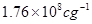Ans4. From Millikan’s experiment, the charge of an electron (e) is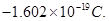Ans5. mass of an electron (m) =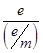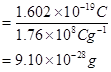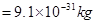So, the mass of an electron isor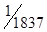th of the mass of a hydrogen atom.
Ans6. (i) The mass of a proton is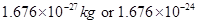(ii) The charge of a proton is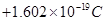Ans7. (i) The mass of a neutron is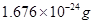(ii) Neutron is electrically neutral i.e. it has no charge as an electron or a proton has.
Ans8. The cathode ray discharge tube experiment performed by J.J. Thomson led to the discovery of negatively charged particles called electron.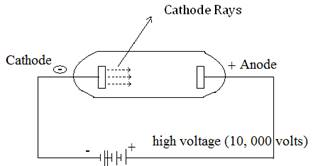A cathode ray tube consists of two thin pieces of metals called electrodes sealed inside a glass tube with sealed ends. The glass tube is attached to a vacuum pump and the pressure inside the tube is reduced to 0.01mm. When fairly high voltage (10, 000V) is applied across the electrodes, invisible rays are emitted from the cathode called cathode rays. Analysis of this rays led to the discovery electrons.
Ans9. The canal ray experiment led to the discovery of –
(i) The anode rays, travel in straight line
(ii) They are positively charged as they get deflected towards the –ve end when subjected to an electric and magnetic field.
(iii) They depend upon the nature of gas present in the cathode tube.
(iv) The charge to mass ration (e/m) of the particle is found to depend on the gas from which they originate.
(v) They are also material particles
The analysis of these proportions led to the discovery of positively charged proton.